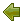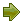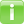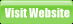Project
Code
Meter

## Weighted Micro Function Points (WMFP)

WMFP is a modern software sizing algorithm invented by Logical Solutions in 2009 which is a successor to solid ancestor scientific methods as COCOMO, Maintainability Index, produces more accurate results than traditional software sizing tools, while requiring less configuration and knowledge from the end user, as most of the estimation is based on automatic measurements of an existing source code.
As many ancestor measurement methods
use source Lines Of Code (LOC)  to measure software size, WMFP uses a parser to understand the source code breaking it down into micro functions and derive several code complexity and volume metrics, which are then dynamically interpolated into a final effort score.

### Measured Elements

The WMFP measured elements are several different metrics deduced from the source code by the WMFP algorithm analysis. They are represented as percentage of the whole unit (project or file) effort, and are translated into time. ProjectCodeMeter displays these elements both in units of absolute minutes and in percentage of the file or project, according to the context.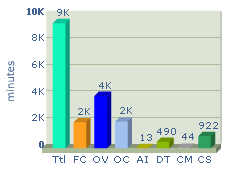Flow Complexity (FC) - Measures the complexity of a programs' flow control path in a similar way to the traditional Cyclomatic Complexity, with higher accuracy by using weights and relations calculation.
Object Vocabulary (OV) - Measures the quantity of unique information contained by the programs' source code, similar to the traditional Halstead Vocabulary with dynamic language compensation.
Object Conjuration (OC) - Measures the quantity of usage done by information contained by the programs' source code.
Arithmetic Intricacy (AI) - Measures the complexity of arithmetic calculations across the program
Data Transfer (DT) - Measures the manipulation of data structures inside the program
Code Structure (CS) - Measures the amount of effort spent on the program structure such as separating code into classes and functions
Inline Data (ID) - Measures the amount of effort spent on the embedding hard coded data
Comments (CM) - Measures the amount of effort spent on writing program comments

### Calculation

The WMFP algorithm uses a 3 stage process: Function Analysis, APPW Transform, and Result Translation.
as shown in the following diagram: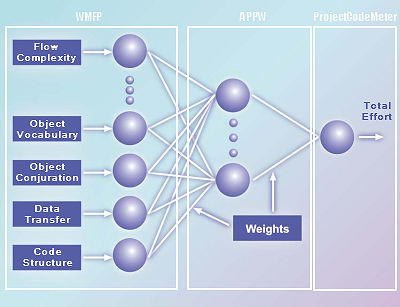A dynamic algorithm balances and sums the measured elements and produces a total effort score.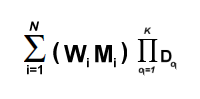M = the Source Metrics value measured by the WMFP analysis stage
W = the adjusted Weight assigned to metric M by the APPW model
N = the count of metric types
i = the current metric type index (iteration)
D = the cost drivers factor supplied by the user input
q = the current cost driver index (iteration)
K = the count of cost drivers

This score is then transformed into time by applying a statistical model called Average Programmer Profile Weights (APPW) which is a proprietary successor to COCOMO II 2000 and COSYSMO. The resulting time in Programmer Work Hours is then multiplied by a user defined Cost Per Hour of an average programmer, to produce an average project cost, translated to the user currency.